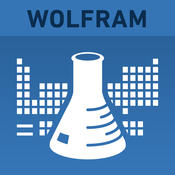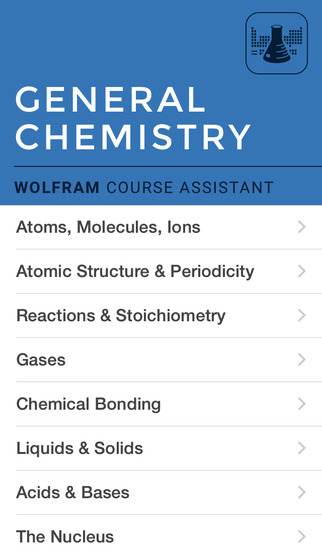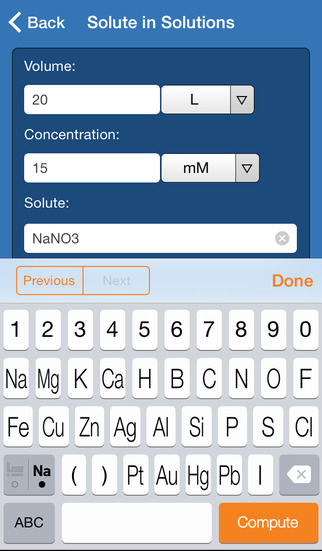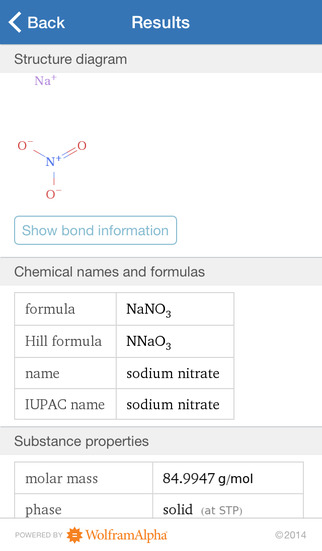5.0

# Wolfram General Chemistry Course Assistant

• 内容摘要
Taking introductory chemistry, or need a quick chemistry reference? Then you need the Wolfram General Chemistry Course Assistant. This comprehensive app for chemistry will help you quickly solve your homework problems, ace your tests, and quiz your understanding of basic chemistry concepts so you're prepared for your courses. Forget canned examples! The Wolfram General Chemistry Course Assistant solves your specific chemistry problems on the fly.

This app covers the following topics applicable to Introductory Chemistry, General Chemistry, and AP Chemistry:

- Determine properties of elements, chemicals, and ions
- Compute properties of gases using various gas laws
- Perform stoichiometric conversions for solutions
- Balance equations
- Compute the enthalpy, equilibrium constant, and theoretical yield of a reaction
- Perform over 45 different computations

The Wolfram General Chemistry Course Assistant is powered by the Wolfram|Alpha computational knowledge engine and is created by Wolfram Research, makers of Mathematica—the world's leading software system for mathematical research and education.

The Wolfram General Chemistry Course Assistant draws on the computational power of Wolfram|Alpha's supercomputers over a 3G, 4G, or Wi-Fi connection.

展开↓

• 版本中的新功能

展开↓

• 应用截图••#### 同类热门推荐

••AnkiMobile Flashcards 2.0.64 | 30.10MB
•••Toca Life: Hospital 1.0.1 | 220.00MB

#### 开发商的其他应用

•WolframAlpha 1.7.3 | 30.40MB
••••#### 不可错过# 安装同步助手后能更便捷的下载此应用• 免费下载游戏和应用
• 快速安装APP
• 便捷管理、优化手机
A# 连接设备至同步助手，自动安装“Wolfram General Chemistry Course Assistant”

### 安装步骤• 色情内容
• 赌博
• 暴力内容
• 反动内容
• 侵犯版权
• 木马病毒
• 恶意扣费
• 不良插件
• 无法安装或者启动
• 其他